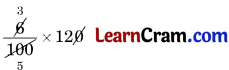# DAV Class 6 Maths Chapter 5 Worksheet 2 Solutions

The DAV Class 6 Maths Solutions and DAV Class 6 Maths Chapter 5 Worksheet 2 Solutions of Percentage and its Applications offer comprehensive answers to textbook questions.

## DAV Class 6 Maths Ch 5 WS 2 Solutions

Question 1.
Find:

(a) The amount 6% more than ₹ 120
Solution:
6% of ₹ 120
== $$\frac{36}{5}$$
= ₹ 7.20
Amount more than ₹ 120 = ₹ 120 + ₹ 7.20
= ₹ 127.20
Hence, the required amount = ₹ 127.20.

(b) The amount 15% less than ₹ 400
Solution:
15% of ₹ 400
= $$\frac{15}{100}$$ × 400
= ₹ 60
∴ The amont less than ₹ 400
= ₹ 400 – ₹ 60 = ₹ 340
Hence, the required amount = ₹ 340

Question 2.
The monthly salary of Mohan is ₹ 950. If his salary increases by 6%, find his new salary.
Solution:
Monthly salary of Mohan = ₹ 950
Increase in his salary = 6 % of ₹ 950
== ₹ 57
∴ His new salary = ₹ 950 + ₹ 57 = ₹ 1007
Hence, the required salary = ₹ 1007

Question 3.
A dealer buys an old Car for ₹ 80,000. He repairs it and sells it at an increase of 10%. What is the selling price of the Car?
Solution:
Price of the Car = ₹ 80,000
Increased price after repairing the Car = $$\frac{10}{100}$$ × 80,000
= ₹ 8,000
∴ The increased price = ₹ 80,000 + ₹ 8000 = ₹ 88000
Hence, the required selling price = ₹ 88,000

Question 4.
The monthly consumption of milk in a family in 120 litres. If due to festival, the consumption of milk increased by 20%, find the present consumptions of milk.
Solution:
Monthly consumption of milk = 120 litres
Increased consumption = $$\frac{20}{100}$$ × 120
= 24 litre
∴ Present consumption = 120 litres + 24 litres = 144 litres
Hence, the required consumption of milk = 144 litres.

Question 5.
The cost of a saree was ₹ 500. If the shopkeeper allows a discount of 20% on the saree, what is the cost of saree after discount?
Solution:
Cost of saree = ₹ 500
Discount = 20% of ₹ 500
= $$\frac{20}{100}$$ × 500
= ₹ 100
∴ Cost of saree after the discount = ₹ 500 – ₹ 100 = ₹ 400
Hence, the required cost = ₹ 400.